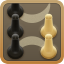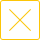# 24点必考100道题(包含数字3的24点题目及答案)

24点必考100道题，包含数字3的24点题目及答案。24点休闲小游戏是常见的一种扑克牌休闲游戏玩法，常见玩法为从一副扑克牌中抽取四张数字牌，运营乘、除、加、减，每个数字用一次，最后计算结果等于24，下面文章中列出了包含数字3的24点题目及答案，其他点数可以前往同城游资讯栏目查看更多。3333：3*3*3-3=24

3334：3+3*(3+4)=24

3335：3*3+3*5=24

3336：3+3+3*6=24

3337：3*(3/3+7)=24

3338：(3+3-3)*8=24

3339：3*(9-3/3)=24

3344：3*(3*4-4)=24

3345：4*(3/3+5)=24

3346：(3-3+4)*6=24

3347：3*(4-3+7)=24

3348：(8-4)*(3+3)=24

3349：3+9+3*4=24

3355：5*5-3/3=24

3356：3+6+3*5=24

3357：3*(3*5-7)=24

3359：(9-5)*(3+3)=24

3366：3*(6/3+6)=24

3367：3*(3+7)-6=24

3368：(3*3-6)*8=24

3369：9+6+3*3=24

3377：(3/7+3)*7=24

3378：8+7+3*3=24

3379：3+7*9/3=24

3388：8/(3-8/3)=24

3389：3*(3+8)-9=24

3399：9+3+3+9=24

3444：4*(3+4)-4=24

3445：3+5+4*4=24

3446：(4/4+3)*6=24

3447：3*(4/4+7)=24

3448：(3+4-4)*8=24

3449：3*(9-4/4)=24

3455：5+4+3*5=24

3456：(3+5-4)*6=24

3457：7+5+3*4=24

3458：4*(3+5)-8=24

3459：3*(4+9-5)=24

3466：6+6+3*4=24

3468：(3*4-8)*6=24

3469：4*(3+9-6)=24

3477：3+4*7-7=24

3478：8+(7-3)*4=24

3479：3*(4+7)-9=24

3489：9+3+4+8=24

3499：9+3*(9-4)=24

3556：(5/5+3)*6=24

3557：3*(5/5+7)=24

3558：(3+5-5)*8=24

3559：3*(9-5/5)=24

3566：(3+6-5)*6=24

3567：3*(6-5+7)=24

3568：3*(6-5)*8=24

3569：3+5*6-9=24

3578：8+3*7-5=24

3579：9+7+3+5=24

3588：8+3+5+8=24

3589：5+3*9-8=24

3599：9+5*9/3=24

3666：(6/6+3)*6=24

3667：3*(6/6+7)=24

3668：(3+6-6)*8=24

3669：9+6+3+6=24

3677：3*(7-6+7)=24

3678：8+7+3+6=24

3679：3*(6+9-7)=24

3688：(8/8+3)*6=24

3689：(3+9-8)*6=24

3699：6+3*9-9=24

3777：7+7+3+7=24

3778：(3+7-7)*8=24

3779：3*(9-7/7)=24

3788：3*(8/8+7)=24

3789：3*(7+9-8)=24

3799：3*(9/9+7)=24

3888：(3+8-8)*8=24

3889：(9-8)*3*8=24

3899：9+3*8-9=24

3999：3*(9-9/9)=24

## 精品排行

1189759人在玩 2
###### 常州四副牌​（四副头）
23201人在玩 3
###### 双陆棋
3## 同城游-本地棋牌游戏合集热门攻略更多